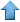Precision RecruitingData Mining Contest Math Jobs * Site Map
 [ Home ] [ Finance ] [ Web Audit ] [ Consulting ]

These are difficult mathematical questions. They are arising from real applications such as fraud detection, arbitrage and scoring systems. If you have interesting answers to any questions, feel free to email us your comments or solution. The best answers will be published here. Companies and Organizations interested in submitting problems should E-mail us.

Iterative Algorithm for Linear Regression

I am trying to solve the regression Y=AX where Y is the response, X the input, and A the regression coefficients. I came up with the following iterative algorithm:

Ak+1 = cYU + Ak (I-cXU),
where:
1. c is an arbitrary constant
2. U is an arbitrary matrix such that YU has same dimension as A. For instance U = transposed(X) works.
3. A0 is the initial estimate for A. For instance A0 is the correlation vector between the independent variables and the response.
Questions:
1. What are the conditions for convergence? Do I have convergence if and only if the largest eigenvalue (in absolute value) of the matrix I-cXU is strictly less than 1?
2. In case of convergence, will it converge to the solution of the regression problem? For instance, if c=0, the algorithm converges, but not to the solution. In that case, it converges to A0.
Parameters:
1. n: number of independent variables
2. m: number of observations
Matrix dimensions:
1. A: (1,n) (one row, n columns)
2. I: (n,n)
3. X: (n,m)
4. U: (m,n)
5. Y: (1,m)
1. We are dealing with an ill-conditioned problem; most independent variables are highly correlated.
2. Many solutions (as long as the regression coefficients are positive) provide a very good fit, and the global optimum is not that much better than a solution where all regression coefficients are equal to 1.
3. The plan is to use an iterative algorithm to start at iteration #1 with an approximate solution that has interesting properties, then move to iteration #2 to improve a bit, then stop.
Note: this question is not related to the ridge regression algorithm described here.

Contributions:

• From Ray Koopman

No need to apologize for not using "proper" weights. See

Dawes, Robyn M. (1979). The robust beauty of improper linear models in decision making. American Psychologist, 34, 571-582.Data Mining • Machine Learning • Analytics • Quant • Statistics • Econometrics • Biostatistics • Web Analytics • Business Intelligence • Risk Management • Operations Research • AI • Predictive Modeling • Actuarial Sciences • Statistical Programming • Customer Insight • Data Modeling • Competitive Intelligence • Market Research • Information Retrieval • Computer Science • Retail Analytics • Healthcare Analytics • ROI Optimization • Design Of Experiments • Scoring Models • Six Sigma • SAS • Splus • SAP • ETL • SPSS • CRM • Cloud Computing • Electrical Engineering • Fraud Detection • Marketing Databases • Data Analysis • Decision Science • Text Mining